## 11973 Reputation

16 years, 56 days

## piecewise...

Replace the if statements with a nested piecewise.

piecewise returns unevaluated if x,y,z are not concrete.

azimuth:=piecewise(x>0,arctan(y/x),x<0,arctan(y/x)+Pi,x=0,piecewise(y>0,Pi/2,y=0,0,y<0,-Pi/2));

## Changes in convert/Vector in Maple 2020...

In Maple 2020 convert/Vector has been changed some:
Try
showstat(convert/Vector);

in earlier versions and compare with Maple 2020.

Immediately noticable is that the number of lines has basically doubled.

Let xxx be anything else than NULL, column or row.

In previous versions convert([1,2,3], xxx) ends up returning Vector[xxx]([1,2,3]), which results in the same error as the following does:

Vector[xxx]([1,2,3]);

In Maple 2020 the code for convert/Vectorhas in line 10 the following statement:
R := if(dir = NULL,Vector['column'](V),~Vector[dir](V));

which causes the problem.
Try in a new session:

restart;
dir:=xxx:
V:=[1,2,3];
R := if(dir = NULL,Vector['column'](V),~Vector[dir](V));


You will loose the kernel.

## Added later:  If you remove the "coercer" ~ from ~Vector[dir](V) above, you don't loose the kernel, but just get the appropriate error:

Error, (in Vector[xxx]) row or column expected for Vector index

This simple use of ~Vector causes a loss of kernel connection (in any Maple version from Maple 16 and up:
~Vector[xxx]([1,2,3]);

causes loss of kernel connection.

## No exact solution...

Surely you won't get anything useful out of trying to find an exact solution.

Using the values of R at eta = 0 you could get a series approximation that would (should) resemble the solution in a right-sided neighborhood of 0:

### For convenience in finding ics I use operator output in dsolve:
Digits:=15:
R := dsolve({bc, eq1, eq2}, numeric, output = operator,abserr=1e-12);
ics := R(0)[2 .. -1][];
S:=dsolve({eq1,eq2,ics},fcns,type=series,order=10);
Sp:=convert(S,polynom);
###
odeplot(R, [eta, f(eta) - eval(f(eta), Sp)], 0 .. 1); # OK on that short interval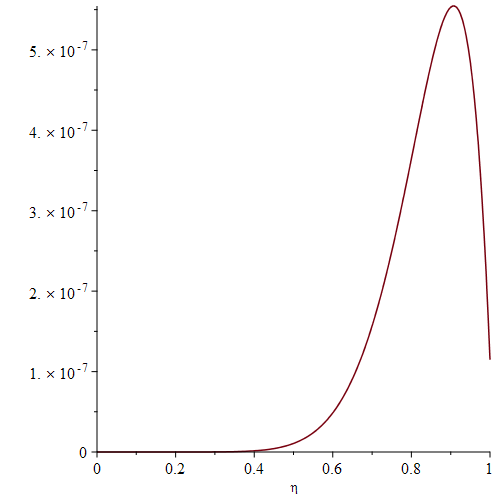######################################

An even better idea could be to solve the initial value problem using the ics we found from solving the bvp. Then find the differences. It should be noticed that the algorithms for solving bvp and ivp are quite different.

R_init:=dsolve({ics, eq1, eq2}, numeric, abserr=1e-12,relerr=1e-10,output=operator);
F,F1,F2,G,G1,G2:=op(rhs~(R_init[2..-1]));
odeplot(R,[eta,f(eta)-F(eta)],0..N);
odeplot(R,[eta,g(eta)-G(eta)],0..N);
odeplot(R,[eta,diff(f(eta),eta)-F1(eta)],0..N);
### Similarly with the other derivatives.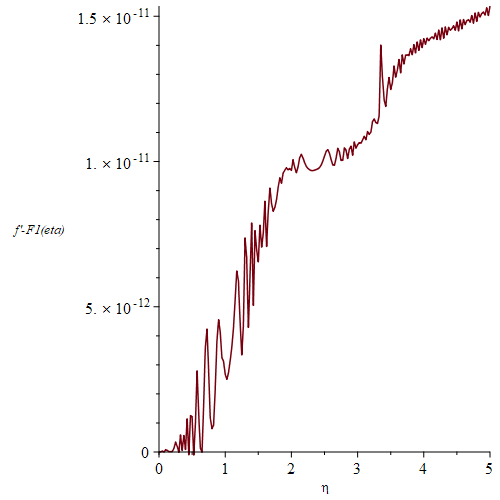## An improved version...

@Alger As suggested by Dr. Venkat Subramanian I have added implicit=true and optimize=true.

Besides that I have made a procedure that on input of the value of C and the range of t-values computes the solution.

This is convenient when different C's are considered.

Computing times are much improved. A "singularity" is still announced for C = 0.000045 and range 0..100, but this time it doesn't happen till t = 81.598332, which again makes me distrust the existence of a singularity.

MaplePrimes20-04-30_odesystemIII.mw

Using method=ck45 (i.e. Cash-Karp) instead of the default rkf45 we don't get any complaints for C=0.000045 and range = 0..100:
With the procedure res from the uploaded worksheet do:

VARS:=[[t,ids(t)],[t,ipdr(t)],[t,ipqr(t)],[t,iqs(t)],[t,vds(t)],[t,vqs(t)]];
dsol_45:=res(0.000045,0..100,method=ck45);
odeplot(dsol_45,VARS,0..100,numpoints=10000);
odeplot(dsol_45,VARS[-2..-1],99.9..100,numpoints=1000);


The plots: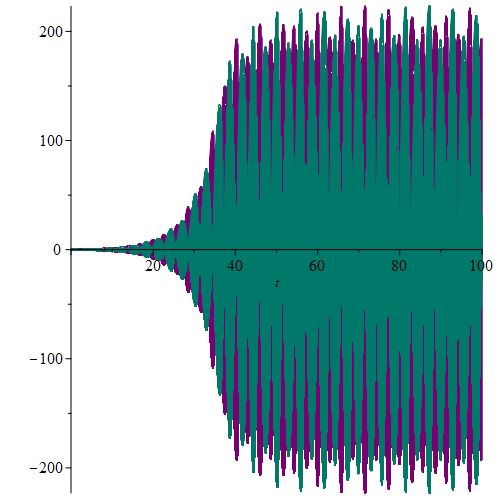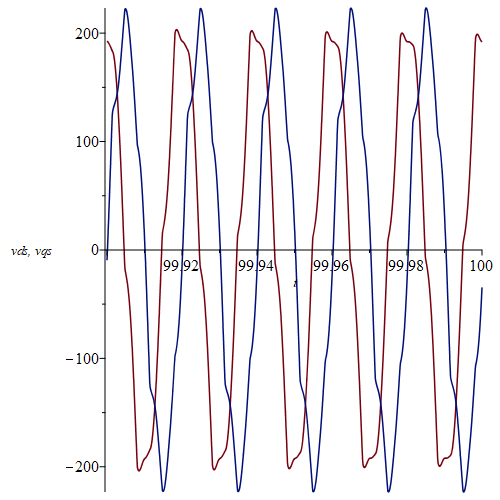#################################

Here is a slightly revised procedure res (called res2) which doesn't require the range argument, but allows it. The intention is to find the approximate period of a potentially periodic solution.

#Allowing for leaving out the range simply by letting it not be a required parameter.
#It can be added in the form range= t0..t1 in the call to res2 if desired.
res2:= proc(c::realcons) global C;
dsolve(eval({eq1, eq2, eq3, eq4, ep5, ep6, ics},C=c), numeric,
maxfun = 10^8,compile=true,implicit=true,optimize=true,_rest)
end proc;
#Here the intention is to find the approximate period of a potentially periodic solution. We use #zeros of vds.
#The distance between two consequtive zeros times 2 should be the period in this case.
#We only take note of zeros beyond t=99.8 and up to 100.
dsol_45E2:=res2(0.000045,method=ck45,events=[[[vds(t),t>99.8],none]]);
dsol_45E2(99.805); # This first call takes a while
T1:=op(dsol_45E2(eventfired=)); # The event fired at this time
dsol_45E2(99.815); # This is fast because it starts from the result above.
T2:=op(dsol_45E2(eventfired=)); # The next zero
#Now automating this using the results T1 and T2:
A:=Array(datatype=float):
A(1):=T1:
A(2):=T2:
d:=T2-T1: eps:=1e-4: # eps = tolerance
for i from 3 while T2<=100 do
dsol_45E2(T2+d+eps);
T1:=T2;
T2:=op(dsol_45E2(eventfired=));
A(i):=T2;
d:=T2-T1
end do:

S:=[seq(A[i+1]-A[i],i=1..numelems(A)-1)]; # The consequtive differences between zeros
listplot(S);
wr; # The period of sin(wr*t) is 2*Pi/wr
2*Pi/wr;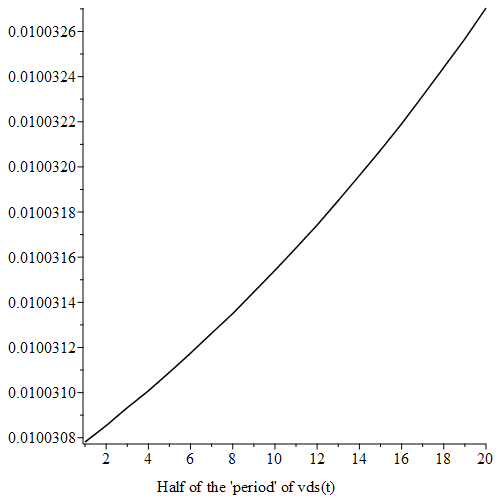## odeplot...

Why not use odeplot instead of plot in the last plots too?

Here I take the code from acer's answer:

CodeTools:-Usage(odeplot(dsol1,[t,(cos(wr*t)*vds(t) - sin(wr*t)*vqs(t))*sqrt(3)], 0 .. 40, labels=[t,'Uas'], color = ["Red"], style = [line],numpoints = 7500));
###
CodeTools:-Usage(odeplot(dsol1,[t,(cos(wr*t)*ids(t) - sin(wr*t)*iqs(t))], 0 .. 40, labels=[t,'Ias'], color = ["Red"], style = [line],numpoints = 7500));


## solve...

Using solve:

restart;
eqs:=(-(2*I)*c1*eta+c1*sqrt(-e0^2-4*eta^2))/e0 = c2, ((2*I)*c2*eta+c2*sqrt(-e0^2-4*eta^2))/e0 = -c1;
solve({eqs},{c1,c2});


We see that c2 can be anything (c2=c2) and then if c2 is chosen, c1 is determined.

## Two variables only...

Go to ?pdsolve,numeric and you will find that the help page for pdsolve/numeric in the beginning in the section Parameters says:

PDEsys-single or set or list of time-dependent partial differential equations in two independent variables

Thus even if your equation had no integral pdsolve/numeric couldn't handle it.

## uneval modifier...

If I understand you correctly, what you could use is the uneval modifier in your procedure.

Here is the code for simplify2 with a few changes:

restart;

simplify2 := proc (Term1::uneval)
# In some cases the term gets longer after the simplification. Then discard.
# Give the local procedure variable a long name. They must not be identical in Term.
local simplify_tmpvar_c1, simplify_tmpvar_c1sum, simplify_tmpvar_c2, \
simplify_tmpvar_c2sum, simplify_tmpvar_nms, simplify_tmpvar_k, Term2,Term: #Term local now
# Assume all contents of the Term to be local variables to this procedure
# Otherwise, variables like "l1" can be overwritten by occurences in the calling worksheet.
Term:=eval(Term1,2); ############# change
simplify_tmpvar_nms:=convert(indets(Term,name),list): # get list of symbols
for simplify_tmpvar_k from 1 to LinearAlgebra:-ColumnDimension(simplify_tmpvar_nms) do
if simplify_tmpvar_nms[simplify_tmpvar_k] = Pi or simplify_tmpvar_nms[simplify_tmpvar_k] = 0 then
next: # 0 and Pi can not be variables
end if:
eval(sprintf("local %s;", String(simplify_tmpvar_nms[simplify_tmpvar_k]))); # assume local
end do:

# Get computational effort before simplification
simplify_tmpvar_c1sum := diff(simplify_tmpvar_c1,functions)+ \
diff(simplify_tmpvar_c1,multiplications)+\
diff(simplify_tmpvar_c1,divisions):
# Perform simplification. Attention: tries to use global variables to simplify the expression (see above)
Term2 := simplify(Term):
# Get effort after simplification
simplify_tmpvar_c2sum := diff(simplify_tmpvar_c2,functions)+\
diff(simplify_tmpvar_c2,multiplications)+\
diff(simplify_tmpvar_c2,divisions):
if simplify_tmpvar_c2sum > simplify_tmpvar_c1sum then
# The simplification was not successful. Take old version.
Term2 := Term:
end if:
return Term2:
end proc:
#with(LinearAlgebra): with(ArrayTools): with(codegen): with(CodeGeneration): with(StringTools):

T := m2*(l1+l2)*(qD2)^2; # dummy-expression for kinetic energy
l1 := length(T);
T;
T_simpl := simplify2(T);

T_simpl;
l2 := length(T_simpl);


## A way...

res:=[solve](1/2*m*v^2 = 1/2*F*x, v);
res1:=simplify(res) assuming positive;


## Stepwise...

Although I do think that the exact solver in this piecewise case should do this problem correctly, it can be done manually as I have here.
It should be noted that due to a bug in Maple 2020 this only works in previous versions (well at least in Maple 2019.2 and 2018.2, and also in the one you are using Maple 2015):

restart;
with(plots):
Digits:=20:
perturbation := .9*(diff(epsilon(t), t, t)) = (-28.67085587*piecewise(0.2000000000e-1*t < 0.8e-1, 0., 0.2000000000e-1*t < .12, -0.8e-1+0.2000000000e-1*t, 0.2000000000e-1*t < .14, .16-0.2000000000e-1*t, 0.2e-1)-0.7645561571e-1*t-1.548363347)*(diff(epsilon(t), t))-0.7596363e-1*piecewise(0.2000000000e-1*t < 0.8e-1, 0., 0.2000000000e-1*t < .12, -0.8e-1+0.2000000000e-1*t, 0.2000000000e-1*t < .14, .16-0.2000000000e-1*t, 0.2e-1)-0.202569683e-3*t+0.26997403e-1-10.10*epsilon(t)
P:=simplify(perturbation,piecewise);
ivp:={P,epsilon(t0)=e0, D(epsilon)(t0)=e0_1}; # For general use
ivp0:=eval(ivp,{t0=0,e0=0,e0_1=0}) assuming t<4;
EPS1:=rhs(dsolve(ivp0));
e4,e4_1:=op((evalf@eval)~([EPS1,diff(EPS1,t)],t=4));
e4,e4_1:=op(simplify(fnormal([e4,e4_1]),zero));
ivp4:=eval(ivp,{t0=4,e0=e4,e0_1=e4_1}) assuming t>=4,t<6;
EPS2:=rhs(dsolve(ivp4));
plot(piecewise(t<4,EPS1,EPS2),t=0..6);
e6,e6_1:=op((evalf@eval)~([EPS2,diff(EPS2,t)],t=6));
e6,e6_1:=op(simplify(fnormal([e6,e6_1]),zero));
ivp6:=eval(ivp,{t0=6,e0=e6,e0_1=e6_1}) assuming t>=6,t<7;
EPS3:=rhs(dsolve(ivp6));
plot(piecewise(t<4,EPS1,t<6,EPS2,EPS3),t=0..7);
e7,e7_1:=op((evalf@eval)~([EPS3,diff(EPS3,t)],t=7)); # No small imaginary parts
ivp7:=eval(ivp,{t0=7,e0=e7,e0_1=e7_1}) assuming t>=7;
EPS4:=rhs(dsolve(ivp7));
EPS:=piecewise(t<4,EPS1,t<6,EPS2,t<7,EPS3,EPS4): # The solution
plot(EPS,t=0..10,color=blue); pexact:=%:
### Now numerically:
numsol := dsolve({perturbation, epsilon(0)=0, D(epsilon)(0)=0}, numeric,abserr=1e-20,relerr=1e-18,maxfun=0):

odeplot(numsol, [t, epsilon(t)], t=0..10, gridlines=true, color=red); pnumeric:=%:
display(pexact,pnumeric); # No difference discernible
odeplot(numsol,[t, epsilon(t)-EPS],0..10); # The difference


Note:  The problem in Maple 2020 shows up here:

EPS3:=rhs(dsolve(ivp6));
Error, (in dsolve) invalid left hand side of assignment

## frontend...

frontend does a good job here. Using the example by Tom Leslie (resembling yours):

restart;
expr:=(diff(P(t),t)+sin(Pi*x/L)*a(t))/(L^4*k*A*G)+sin(Pi*x/L)*diff(a(t),t\$4)+sin(Pi*x/L)*diff(P(t),t);
####
frontend(coeff,[expr,a(t)]);
frontend(coeff,[expr,diff(P(t),t)]);
frontend(coeff,[expr,sin(Pi*x/L)]);


## The loop...

The "end do" in the loop should clearly be at the end.
So with that corrected your code runs:

restart;
with(plots):
with(plottools):
K := 48;
R1 := 30; R2 := 20; OA := R1+R2;
Vodilo := PLOT3D(cuboid([-3, -3, 0], [OA+4, 4, 2]));
Koleso1 := PLOT3D(cylinder([0, 0, 5], R1, 18), cylinder([0, 0, -2], 1, 16));
Koleso2 := PLOT3D(cylinder([0, 0, 7], R2, 6), cylinder([0, 0, -2], 1, 16));
Amplituda := .3:
###
for i from 0 to K do
phi := sin(6.28*i/K)*Amplituda;
phi1 := phi*(R1+R2)/R2;
P1 := rotate(Koleso2, 0, 0, -phi1);
P2 := rotate(Vodilo, 0, 0, -phi);
x := OA*cos(phi);
y := OA*sin(phi);
P[i] := display(Koleso1, translate(P1, x, y, 0), P2)
end do: # colon
###

display(seq(P[i], i = 0 .. K), orientation = [55, 101], insequence = true, scaling = constrained, axes = normal);


## Maybe this...

This unassigns all the numbered members:

restart;
with(Statistics):
X := a -> RandomVariable(Exponential(a)):
##
for n from 1 to 100 do
S := X(n);
# here are some operations to do
end do:
##
A:={anames(alluser)}:
IA:=indets(A,suffixed(_ProbabilityDistribution,integer)):
##
eval(_ProbabilityDistribution5);
exports(_ProbabilityDistribution5);
##
unassign(op(IA));
eval(_ProbabilityDistribution5);


But you might want to unassign all but the last one:

N:=100:
for n from 1 to N do
S := X(n);
# here are some operations to do
if n = N-1 then
A:={anames(alluser)};
IA:=indets(A,suffixed(_ProbabilityDistribution,integer));
unassign(op(IA));
end if
end do:
anames(alluser);


## Infinitely many solutions...

Your integral equation has infinitely many solutions because the corresponding homogeneous equation has a one parameter family of solutions.
A particular solution can be found as vv did.

You will find my reasoning in the attached worksheet:

Preben Alsholm, March 17 2020.

 > restart;

Notice that I have replaced the coefficient 1/2 of the integral with the parameter a:

 > eq := Z(x) =  3/2-9/2*exp(-2*x)-9/2*exp(-x)+a*int(exp(-y)*Z(x-y), y= 0..ln(2));
 > EQ:=IntegrationTools:-Change(eq, x-y=t, t);

The corresponding homogeneous equation:

 > EQ0:=Z(x) = a*Int(exp(t - x)*Z(t), t = x - ln(2) .. x);

We find a particular solution to the inhomogeneous equation following vv:

 > ZZ:=x -> A*exp(-2*x)+B*exp(-x)+C;
 > expand(eval( (lhs-rhs)(EQ), Z=ZZ));
 > coeffs(%, [exp(x),exp(2*x)]);
 > ABC:=solve([%],{A,B,C});
 > Zsol:=eval(ZZ(x),ABC);
 > simplify(eval( (lhs-rhs)(EQ), Z=unapply(Zsol,x))); # Check

Now find solutions of the homogeneous equation by first differetiating it and thereby getting a delay differential equation:

 > INT:=combine(-1*(rhs=lhs)(EQ0)); # For use below
 > dde01:=(combine@expand)(diff(EQ0,x));

The delay differential equation:

 > dde0:=eval(dde01,INT);

The equation dde0 has as one solution Z(x) = exp(-x) for all values of a:

 > eval((lhs-rhs)(dde0),Z=(x->exp(-x)));
 > simplify(%);

but notice that Z(x) = exp(-x)  doesn't satisfy  the homogeneous integral equation unless a = 1/ln(2):

 > eval((lhs-rhs)(EQ0),Z=(x->exp(-x)));
 > factor(value(%));

Trying to find other solutions to dde0 of the form Z(x) = exp(r*x):

 > value(eval((lhs-rhs)(dde0),Z=(x->exp(r*x))));
 > u:=(combine@expand)(%/exp(r*x)); # Needs to be zero
 > eval(u,r=-1);

Numerically we see here that for a < 0 there is one solution for r and for a > 0 two solutions except at (as it turns out) at a = 1/ln(2).

One of the solutions is -1.

 > plots:-animate(plot,[u,r=-8..2,-1..1,labels=[r,"u"]],a=-2..3,frames=100);
 > usol:=expand(solve(u=0,r,AllSolutions));
 > zz:=op(indets(usol,suffixed(_Z,integer)));

We take the two real branches:

 > rm1:=eval(usol,zz=-1);
 > r0:=eval(usol,zz=0);
 > plot([rm1,r0],a=-2..3,color=[red,"Green"],thickness=3,legend=["rm1","r0"]);

The two branches meet at a = 1/ln(2):

 > eval(rm1-r0,a=1/ln(2));

Checking that the solutions Z(x) = exp(rrm1*x) and  Z(x) = exp(r0*x) actually satisfy dde0:

First rm1:

 > rr:=expand(eval((lhs-rhs)(value(dde0)),Z=(x->exp(rm1*x))));
 > simplify(rr);
 > rr:=expand(eval((lhs-rhs)(value(dde0)),Z=(x->exp(r0*x))));
 > simplify(rr) ;

Now checking that they also satisfy EQ0, but remember that actually exp(-x) is not a solution unless a = 1/ln(2):

 > rr:=expand(eval((lhs-rhs)(value(EQ0)),Z=unapply(exp(rm1*x),x)));
 > simplify(rr); # I see!  It should only be zero for 0 < a <= 1/ln(2)

Testing with a = 1/2:

 > evalf(eval(rm1,a=1/2));
 > rrc:=expand(eval[recurse]((lhs-rhs)(value(EQ0)),{a=1/2,Z=unapply(exp(rm1*x),x)}));
 > simplify(%); # 0
 > evalf(rrc);  # 0.
 > rrcn:=normal(rrc);
 > simplify(denom(rrcn));
 > evalf(%); # -0.825...
 > simplify(numer(rrcn));

We look at r0.

 > rr:=expand(eval((lhs-rhs)(value(EQ0)),Z=unapply(exp(r0*x),x)));
 > simplify(rr); # # It should only be zero for 1/ln(2) <= a

We look at a = 1/2:

 > eval(r0,a=1/2);
 > evalf(%);
 > rrc:=expand(eval[recurse]((lhs-rhs)(value(EQ0)),{a=1/2,Z=unapply(exp(r0*x),x)}));
 > simplify(%); # 0
 > rrcn:=normal(rrc);
 > simplify(denom(rrcn));
 > evalf(%); # 0.
 > simplify(numer(rrcn)); # 0

The problem appears to be Maple's ability to show that some quantity is actually zero.

Let us do a similar concrete test of the case a = 3  ( > 1/ln(2) ).

We didn't choose a = 2 because it is rather special: rm1 = -1 and r0 = 0 are found without evalf.

 > evalf(1/ln(2));
 > eval(rm1,a=3);
 > evalf(%);
 > rrc:=expand(eval[recurse]((lhs-rhs)(value(EQ0)),{a=3,Z=unapply(exp(rm1*x),x)}));
 > evalf(rrc); # Float(undefined)* ...
 > rrcn:=normal(rrc);
 > simplify(denom(rrcn));
 > evalf(%); # 0.
 > simplify(numer(rrcn)); #0

We look at r0.

 > eval(r0,a=3);
 > evalf(%);
 > rrc:=expand(eval[recurse]((lhs-rhs)(value(EQ0)),{a=3,Z=unapply(exp(r0*x),x)}));
 > simplify(%); #0
 > rrcn:=normal(rrc);
 > simplify(denom(rrcn));
 > evalf(%); # 9.295 ...
 > simplify(numer(rrcn));

Thus we (believe we) have found the following one parameter family of solutions to the inhomogeneous integral equation EQ:

 > SOL:=Zsol+C*piecewise(a<=0,0,a<=1/ln(2),exp(rm1*x),exp(r0*x));

A direct check:

 > rr:=expand(eval((lhs-rhs)(value(EQ)),Z=unapply(SOL,x))):
 > simplify(%); #  0

An animation in C for a = 1/2 on x = 1.5 .. 3.5:

 > SOL1:=eval(SOL,a=1/2);
 > evalf(SOL1);
 > plots:-animate(plot,[SOL1,x=1.5..3.5],C=-2000..2000,trace=24,caption=typeset("a = ",1/2));

An animation in C for a = 3 and on x = -1 .. 2:

 > SOL2:=eval(SOL,a=3);
 > evalf(SOL2);
 > plots:-animate(plot,[SOL2,x=-1..2],C=-1..1,trace=24,caption="a = 3");
 > ###################################################################################################################

We can single out one of these solutions by imposing an initial condition.

We shall assume that a > 0 from now on, since otherwise the solution SOL has no C:

 > eval(SOL,x=0) assuming a>0;

If Z(0) = 0 is required then C is given by C = C0, where:

 > C0:=solve(%,C);

The solution Z(x) = SOL is then

 > SOL0:=eval(SOL,C=C0) assuming a>0;

If furthermore a = 1/2 the solution is

 > SOL12:=eval(SOL0,a=1/2);
 > plot(SOL12,x=0..10);
 > limit(SOL12,x=infinity);
 > Digits:=15:
 > eps:=1e-5: plots:-animate(plot,[SOL0,x=0..10],a=eps..1/ln(2)-eps);

To find the value of SOL0 for a = 1 we must take the limit:

 > SOL1:=limit(SOL0,a=1);
 > limit(SOL1,x=infinity); # 3
 > plot([SOL1,3],x=0..20,linestyle=[1,3],color=[red,black],caption="a = 1");

The value of SOL0 at 1/ln(2) is found by taking the limit:

 > SOL_ln:=limit(SOL0,a=1/ln(2));
 > L_ln:=limit(SOL_ln,x=infinity); # 3*ln(2)/(-1 + 2*ln(2))
 > evalf(L_ln);
 > plot([SOL_ln,L_ln],x=0..20,linestyle=[1,3],color=[red,black],caption=typeset("a = ",1/ln(2)));
 >
 >## No way...

I have corrected some syntactical errors. But dsolve cannot handle this analytically.

Certainly numerically, though:

restart;
ode:=m*diff(x(t),t,t)= - m * A * sin(2*Pi*f*t) - piecewise( abs(x(t)) < x__max, 0, abs(x(t)) >= x__max, -k*(abs(x(t))-x__max)*signum(x(t))) - diff(x(t), t);
dsolve(ode); #NULL
nms:=indets(ode,And(name,Not(constant))) minus {x,t};
res:=dsolve({ode,x(0)=x0,D(x)(0)=x1},numeric,parameters=[nms[],x0,x1]);
res(parameters=[A=1,m=1,f=1,x__max=2,k=2,x0=0,x1=1]); # Example
plots:-odeplot(res,[t,x(t)],0..20);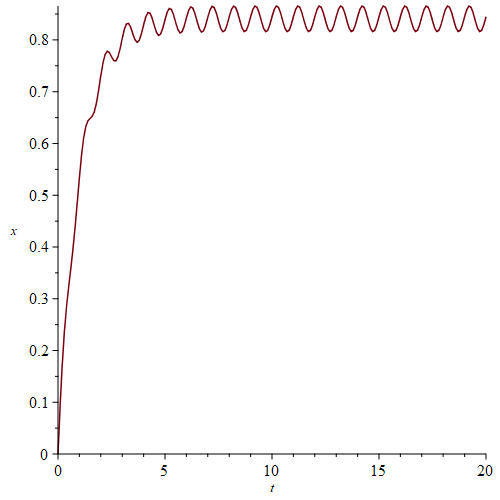﻿